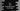# C++ program to find out the missing number in an array## Introduction :

In this tutorial, we will learn how to solve the missing number problem in C++. One array of numbers is given that holds from 1 to n with one number missing in the list. We need to write one program that will find that missing number.

We will write one program to take the array numbers as input from the user. It will ask the user to enter the total numbers of the array excluding the missing number. Next, it will take the numbers as input one by one in increasing order from the user. For example, if the series is 1,2,3,4,5 and if we need to find out 4, the user will enter 1,2,3,5. Then the program will find out the missing number and print it out.

### Algorithm to use :

We are going to use one easy process to solve it. The formula used to find out the sum of all numbers starting from 1 to n is n * (n+1)/2. As we know the value of n, we can find out the sum of all numbers using this formula. We also have all the numbers excluding the missing number. So, we can use one loop and find out the sum of all numbers that the user has entered. The misssing number is the difference between the sum that we have calculated using the formula and the sum of all user input values.

Following are the steps we will use to find the missing number :

1. Find the sum of all numbers in the series of 1 to n using formula.

2. Find the sum of all user input numbers.

3. Find the difference of sum calculated in step 1 and step 2.

### C++ program :

``````#include <iostream>
using namespace std;

int getMissingNumber(int givenArray[], int length)
{
// 5
int sumOfAllNumbers = (length * (length + 1)) / 2;
int sumOfUserNumbers = 0;

for (int i = 0; i < length - 1; i++)
{
sumOfUserNumbers += givenArray[i];
}

//6
int missingNumber = sumOfAllNumbers - sumOfUserNumbers;
return missingNumber;
}

int main()
{
// 1
int length;
cout << "Enter the total count of numbers including the missing number :" << endl;
cin >> length;

// 2
int numArray[length - 1];

// 3
cout << "Enter the numbers excluding the missing number one by one :" << endl;
for (int i = 0; i < length - 1; i++) {
cin >> numArray[i];
}

// 4
cout << "Missing number is : " << getMissingNumber(numArray, length) << endl;
return 0;
}
``````

### Explanation :

The commented numbers in the above program denote the step numbers below :

1. length variable holds the total count of numbers including the missing number. We are reading this data from the user. cin reads the user input value to length.

2. numArray is an integer array used to hold all user provided numbers. It’s size is length - 1. Note that we defined this array only after we got the value of length from the user.

3. In this step, we are reading all numbers excluding the missing number. Inside the for loop, we are reading the numbers and inserting them to the array numArray. This loop runs for length - 1 number of times.

4. Finally, we are printing the missing number. For that, we are calling one method called getMissingNumber. This method finds out the missing number and returns it back. This method takes two parameters : the first one is the user provided array of numbers and the second one is the total count of numbers.

5. This method first calculates the sum of all numbers including the missing number and sum of all numbers that the user has provided i.e. sum excluding the missing number. sumOfAllNumbers variable holds the sum of numbers including the missing number. Here we are using the formula that we discussed above. sumOfUserNumbers holds the sum of all user input numbers. We are using one for loop here to find the sum of user input numbers. The loop iterate through the values of givenArray i.e. the array of user given numbers and adds them all to the variable sumOfUserNumbers.

6. In this step, we are finding the difference of both sum values. The result is stored in the variable missingNumber i.e. the missing number in the array we are looking for. This function returns it back to the main method and we it prints the value on console.

### Sample Output :

Following are a couple of different outputs based on different user inputs :

``````Enter the total count of numbers including the missing number :
5
Enter the numbers excluding the missing number one by one :
1
2
3
4
Missing number is : 5

Enter the total count of numbers including the missing number :
6
Enter the numbers excluding the missing number one by one :
1
3
4
5
6
Missing number is : 2
``````